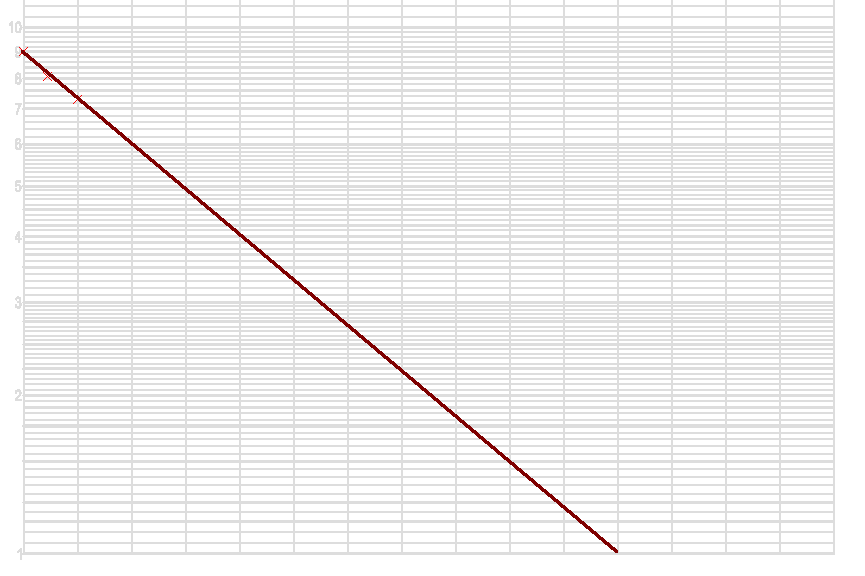SEARCH HOMEMath Central Quandaries & QueriesQuestion from Peter, a student: there is a competition problem where a cottage is worth 25000 years, but it decreases in value every year by 10 percent. Without a calculator, is there a way to find after how many years, the cottage will be worth 2500? (besides multiplying it out and besides logs)Sure Peter. As long as approximation is okay.

Just graph it, ideally using log paper. The situation boils down to (0.9)x = 0.1 Thus you can draw y = (0.9)x on logarithmic graph paper and look for the x value where it crosses y = 0.1.

So I just need one cycle semi-log paper. I know I start at 0.9. The next two are easy: 0.81 and 0.729. I plot these as X marks on the graph, then draw a straight line carefully through them (an exponential on log paper is drawn as a straight line).I see this crosses the 0.1 on the y axis at x = 22, so that means 22 years is the answer to the question. No repetitive calculations (indeed no calculator), no logarithms (except what's built into the graph paper) and a pretty good approximation.

In fact, using logs, I see that x = (log 0.1) / (log 0.9) = 21.85. Not bad.

Hope this helps,
Stephen La Rocque.Math Central is supported by the University of Regina and The Pacific Institute for the Mathematical Sciences.